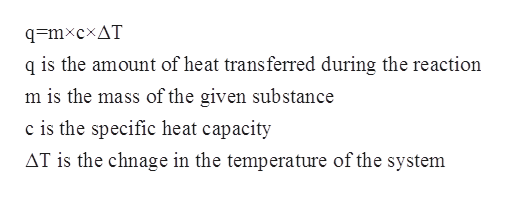# When 3.25 g of sodium hydroxide (NaOH) was dissolved in 150.00 g of water a value of 10.00oC was obtained for ΔT. Calculate the value (calories) for the heat of solution of 3.25 g of NaOH.

Question
220 views

When 3.25 g of sodium hydroxide (NaOH) was dissolved in 150.00 g of water a value of 10.00oC was obtained for ΔT.

Calculate the value (calories) for the heat of solution of 3.25 g of NaOH.

check_circle

Step 1

The specific heat capacity of a substance is the amount of heat which is required to raise the temperature of a given substance per unit of its mass. The relation between specific heat capacity, mass, change in temperature and the heat of the solution is given by the following equation as follows:help_outlineImage Transcriptioncloseq=mxcxAT q is the amount of heat transferred during the reaction m is the mass of the given substance c is the specific heat capacity AT is the chnage in the temperature of the system fullscreen
Step 2

In this problem, it has been given that the mas of sodium hydroxide is 3.25 g and the mass of the water is 150.00 g.

Hence the total mass (m) is as follows:

Step 3

The change in temperature is given to be 10oC  which is equal to 283.15 K.

The specific heat capacity of NaO...

### Want to see the full answer?

See Solution

#### Want to see this answer and more?

Solutions are written by subject experts who are available 24/7. Questions are typically answered within 1 hour.*

See Solution
*Response times may vary by subject and question.
Tagged in

### Chemistry